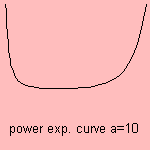# power exponential curve

## power exponential

last updated: 2003-07-20In curve fitting the power exponential curve (you know a better name?) is used for the so-called 'geometric fit'.

The logarithm of the curve is the prime function approximation.

The curve can be generalized to:A = e and b = 1/2 gives the Stirling curve which is an approximation for the factorial 1).Stirling was the one to discover this relation.

notes

1) Often an expression is given as: